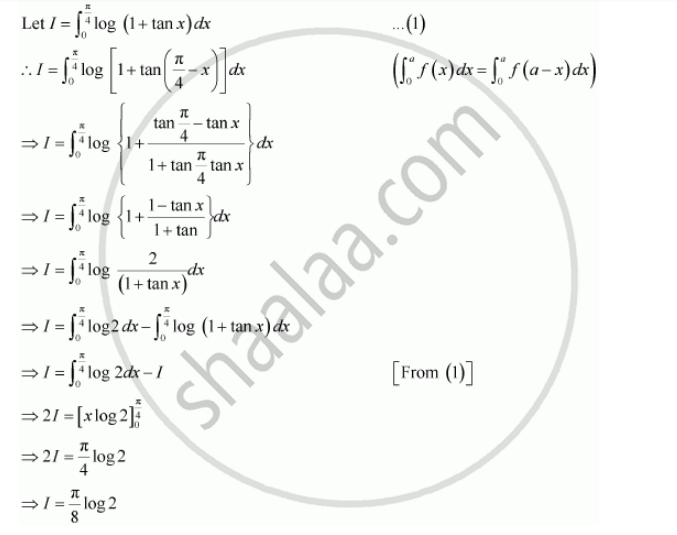Share

Books Shortlist

# By Using the Properties of Definite Integrals, Evaluate the Integrals Int_0^(Pi/4) Log (1+ Tan X) Dx - CBSE (Science) Class 12 - Mathematics

ConceptProperties of Definite Integrals

#### Question

By using the properties of definite integrals, evaluate the integrals

int_0^(pi/4) log (1+ tan x) dx

#### SolutionIs there an error in this question or solution?

#### Video TutorialsVIEW ALL 

Solution By Using the Properties of Definite Integrals, Evaluate the Integrals Int_0^(Pi/4) Log (1+ Tan X) Dx Concept: Properties of Definite Integrals.
S